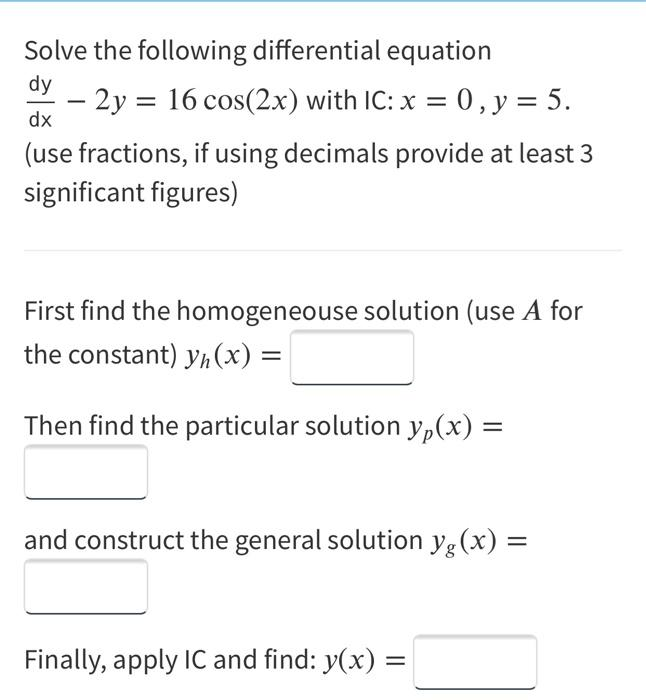### Create an Account

Already have account?

### Forgot Your Password ?

Home / Questions / Solve the following differential equation dy - 2y = 16 cos(2x) with IC: x = 0, y = 5. (use...

# Solve the following differential equation dy - 2y = 16 cos(2x) with IC: x = 0, y = 5. (use fractions, if using decimals provide at least 3 significant figures) dx First find the homogeneouse solution

Solve the following differential equation dy - 2y = 16 cos(2x) with IC: x = 0, y = 5. (use fractions, if using decimals provide at least 3 significant figures) dx First find the homogeneouse solution (use A for the constant) yn(x) = Then find the particular solution yp(x) = and construct the general solution yg(x) = Finally, apply IC and find: y(x) = =Apr 15 2021 View more View Less

#### Answer (Solved)Subscribe To Get Solution